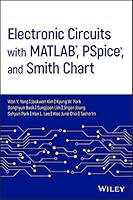# Electronic Circuits with MATLAB, PSpice, and Smith Chart## Book Description

Provides practical examples of circuit design and analysis using PSpice, MATLAB, and the Smith Chart

This book presents the three technologies used to deal with electronic circuits: MATLAB, PSpice, and Smith chart. It gives students, researchers, and practicing engineers the necessary design and modelling tools for validating electronic design concepts involving bipolar junction transistors (BJTs), field-effect transistors (FET), OP Amp circuits, and analog filters.

Electronic Circuits with MATLAB®, PSpice®, and Smith Chart presents analytical solutions with the results of MATLAB analysis and PSpice simulation. This gives the reader information about the state of the art and confidence in the legitimacy of the solution, as long as the solutions obtained by using the two software tools agree with each other. For representative examples of impedance matching and filter design, the solution using MATLAB and Smith chart (Smith V4.1) are presented for comparison and crosscheck. This approach is expected to give the reader confidence in, and a deeper understanding of, the solution. In addition, this text:

• Increases the reader's understanding of the underlying processes and related equations for the design and analysis of circuits
• Provides a stepping stone to RF (radio frequency) circuit design by demonstrating how MATLAB can be used for the design and implementation of microstrip filters
• Features two chapters dedicated to the application of Smith charts and two-port network theory

Electronic Circuits with MATLAB®, PSpice®, and Smith Chart will be of great benefit to practicing engineers and graduate students interested in circuit theory and RF circuits.